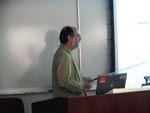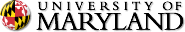Application of the Wigner Distribution to Non-equilibrium Problems at Surfaces: Relaxation, Growth, and Scaling of Capture Zones

CSIC Building (#406), Seminar Room 4122.
Directions: home.cscamm.umd.edu/directions

### Application of the Wigner Distribution to Non-equilibrium Problems at Surfaces: Relaxation, Growth, and Scaling of Capture Zones

Professor Theodore L. EinsteinUniversity of Maryland

Abstract:  The generalized Wigner distribution (GWD), arising from random-matrix theory, has proved amazingly successful at describing the distribution of spacings in a long list of fluctuating systems, including nuclear levels, fermions in 1D, universal conductance fluctuations in disordered wires, intervals between parked cars, and terrace widths on vicinal surfaces.  The GWD has the form Pβ= aβ sβ exp(–bβ s2) [with aβ and bβ being constants assuring normalization and unit mean, and s being the fluctuating quantity divided by its mean.  After a review of these equilibrium situations, we describe three new applications to non-equilibrium problems in surface/interface physics.

1) We develop a Fokker-Plank formalism to describe how steps on a vicinal surface relax to equilibrium (described by the GWD) from an arbitrary initial configuration.

2) We sketch how growth affects the terrace width distribution of a vicinal surface, leading to a narrower distribution, i.e. a GWD with higher β.

3) We discuss in detail applications to the problem of island growth, long-studied numerically but elusive analytically.  Using published kinematic Monte Carlo data, we find the GWD describes well the distribution of the areas of Voronoi polygons (proximity cells) around nucleation centers, i.e. the capture zones (CZ).  In this case, β = i + d/2, where i is the size of the critical nucleus and d the spatial dimensionality. We demonstrate excellent fits of numerical data for both d = 1 and d = 2. To clarify the underlying physics, we present a phenomenological derivation by constructing a Langevin equation similar to that used in deriving the Fokker-Plank equation for relaxation of TWDs. We discuss implications for processing and analysis of experimental data.

Work at UMd supported by the MRSEC, NSF Grant DMR 05-20471, and partially by DOE CMSN grant DEFG0205ER46227. AP's visits to UMd by supported by a CNRS Travel Grant.
[slides]UM Home | Directories | Calendar Maintained by CSCAMM Direct questions and comments to webmaster CSCAMM is part of the College of Computer, Mathematical & Natural Sciences (CMNS)